# Electronics: Circuit Analysis Using Differential Equations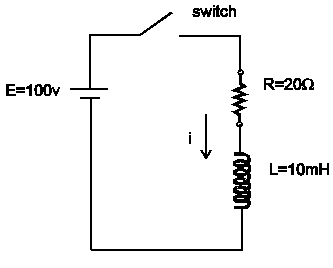## Problem:

Find the current in the circuit to the right after time t=0. The switch is closed at t=0, and the initial current in the circuit is zero.

## Solution:

Using Kirchoff’s Voltage Law:

vL + vR = E

Express the voltage equation in terms of current.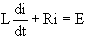Put the above equation in differential form.

L di = (E-Ri) dt

Separate the variables.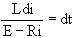Integrate both sides of the equation.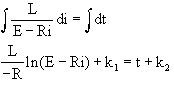Solve for the current i. First isolate the logarithm.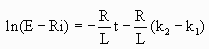Express in exponential form.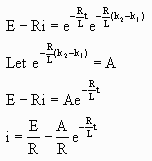Substitute the initial conditions into this equation to evaluate the constant A. The initial conditions are i=0 at t=0.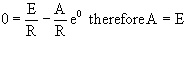Thus the equation becomes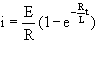Evaluate the expression for the values given in the circuit diagram.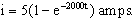A graph of this function is shown below. Note that if the inductor were absent the current would rise instantly to 5 amperes, the value given by ohms law, i=E/R. The presence of the inductor causes the current to rise exponentially to 5 amperes, with the characteristic time being 1/2000 s.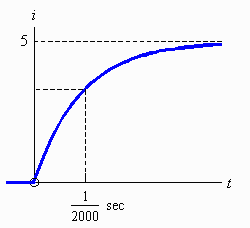Written by Clayton Copping, October 7, 1997